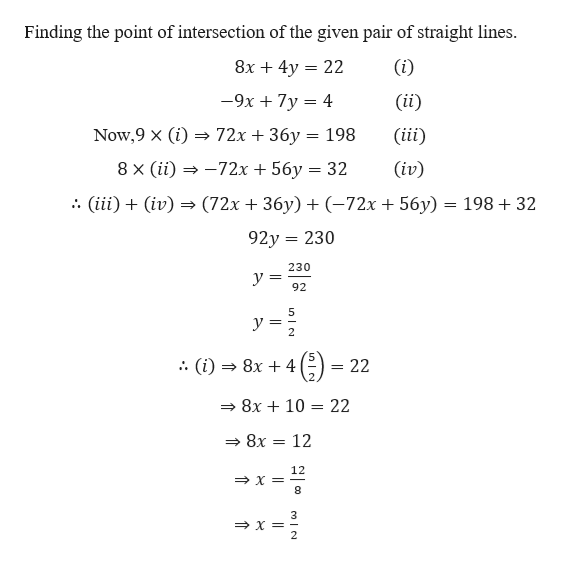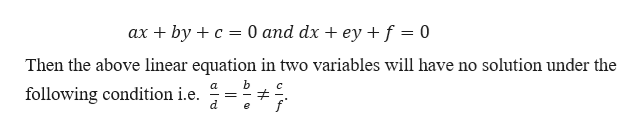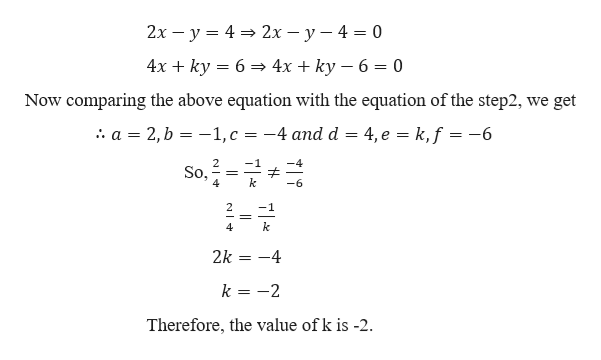# Find the point of intersection of the pair of straight lines.8x + 4y= 22−9x + 7y= 4(x, y) =Determine the value of k for which the system of linear equations2x− y= 44x+ ky= 6has no solution.k =

Question
15 views

Find the point of intersection of the pair of straight lines.

 8x + 4y = 22 −9x + 7y = 4

(x, y) =

Determine the value of k for which the system of linear equations

 2x − y = 4 4x + ky = 6

has no solution.

k =

check_circle

Step 1

Finding the point of intersection of the given pair of straight lines.help_outlineImage TranscriptioncloseFinding the point of intersection of the given pair of straight lines 8x 4y 22 (i) = -9x 7y 4 (ii) 72x + 36y Now,9 X (i) (iii) 198 8 x (ii) 72x56y = 32 (iv) (72x 36y)-72x 56y) = 198+ 32 (ii(iv) 92y 230 230 y 92 5 y 2 5 :. (i) = 22 8x 4 8x +10 = 22 > 8x = 12 12 8 3 X - 2 un l fullscreen
Step 2

We know that,help_outlineImage Transcriptioncloseax byc 0 and dx ey f = 0 Then the above linear equation in two variables will have no solution under the C following condition i.e. d fullscreen
Step 3

Given,

...help_outlineImage Transcriptionclose2x - y42x - y - 4 = 0 4x + ky 6 4x + ky - 6 = 0 Now comparing the above equation with the equation of the step2, we get a 2,b -1,c =-4 and d 4, e = k,f = -6 So, 4 6 2 k 4 2k 4 k -2 Therefore, the value of k is -2 T NI fullscreen

### Want to see the full answer?

See Solution

#### Want to see this answer and more?

Solutions are written by subject experts who are available 24/7. Questions are typically answered within 1 hour.*

See Solution
*Response times may vary by subject and question.
Tagged in

### Equations and In-equations